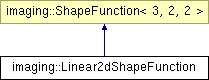# imaging::Linear2dShapeFunction Class Reference [Finite Element Module]

Linear shape function on the triangle. More...

`#include <ShapeFunction.hpp>`

Inheritance diagram for imaging::Linear2dShapeFunction:List of all members.

## Public Member Functions

float_t value (size_t node_index, const ublas::fixed_vector< float_t, 2 > &in) const
ublas::fixed_vector< float_t, 2 > & gradient (size_t node_index, const ublas::fixed_vector< float_t, 2 > &in, ublas::fixed_vector< float_t, 2 > &out) const

## Static Public Member Functions

static size_t face_node (size_t face, size_t node)

## Detailed Description

Linear shape function on the triangle.

Linear shape function on the triangle determined by the vertices (0, 0), (1, 0), (0, 1) (i.e. 3 element nodes). You will rarely use this class directly, but might plug it into your own FE traits class as shape_function_t.

## Member Function Documentation

 size_t imaging::Linear2dShapeFunction::face_node ( size_t face, size_t node ) ` [static]`

Returns the node index (on the element) of the node determined by face_index and face_node_index (on the boundary reference element). E.g. the vertex 1 (there are only vertices 0 and 1 on the boundary reference element) on the face 2 of the square reference element (there are 4 faces with indices from zero to 3) is mapped to vertex 3 on the reference element.

Reimplemented from imaging::ShapeFunction< 3, 2, 2 >.

References imaging::Triangle2dTransform::face_vertex().

 float_t imaging::Linear2dShapeFunction::value ( size_t node_index, const ublas::fixed_vector< float_t, 2 > & in ) const

Returns the value of the shape function corresponding to node_index at in.

 ublas::fixed_vector< float_t, 2 > & imaging::Linear2dShapeFunction::gradient ( size_t node_index, const ublas::fixed_vector< float_t, 2 > & in, ublas::fixed_vector< float_t, 2 > & out ) const

Computes the gradient of the shape function corresponding to node_index at in and stores the result in out.

The documentation for this class was generated from the following files:

Generated on Tue Feb 10 10:01:31 2009 for imaging2 by1.5.5